Latest Banking jobs   »

# Quantitative Aptitude Quiz For RBI Grade B Phase 1 2023 -18th May

Directions (1-5): In the given questions, two quantities are given, one as ‘Quantity I’ and another as ‘Quantity II’ you have to determine relationship between two quantities and choose the appropriate option.
(a) Quantity I > Quantity II
(b) Quantity I < Quantity II
(c) Quantity I ≥ Quantity II
(d) Quantity I ≤ Quantity II
(e) Quantity I = Quantity II or no relation

Q1. Quantity I:– A train can cover 60km in 45 min. The distance covered by train in 3 hours.
Quantity II:– Two trains are moving towards each other with speed of 40 km/h and 80 km/h. They meet after 6 hour journey then difference of distance covered by both the trains.

Q2. Quantity I:– In a class total no. of students are 500. Average marks of boys is 42 and average marks of girls and class are 38, 40 respectively. No of boys in that class.
Quantity II:– Average of five numbers is 375. Average of first two numbers is 150 and average of last two numbers is 650, third number is.

Q3. Quantity I:– A shopkeeper blends two type of Rice costing 28 Rs./kg and 35 Rs./kg and Ratio of quantity of costing 28 Rs./kg to quantity of 35 Rs./kg in mixture is 3 : 4. Average cost per kg of mixture.
Quantity II:– A shopkeeper gives 10% discount and gains 10%. If cost price is Rs 18. His market price is.

Q4. Quantity I:– A square of side 7/2 meter is bent into form of a circle. Area of circle.
Quantity II:– A cylinder of height 7 meter and radius of base is 50 centimeter curved surface area of cylinder is.

Q5. Quantity I:– 14x² – 31x = –12
Value of x.
Quantity II:– 14y² – 13y = –3
Value of y

Directions (6 -7): In the given questions, two quantities are given, one as ‘Quantity I’ and another as ‘Quantity II’. You must determine relationship between two quantities and choose the appropriate option: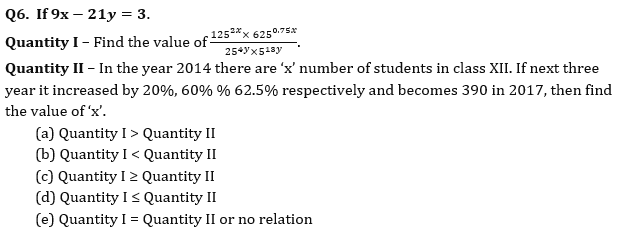Q7. Quantity I – How many four-digit numbers can be formed from digits 0, 2, 4, 6, 5, 1 such that each number is divisible by 5 and no repetition is allowed.
Quantity II – Ratio between 25% of ‘a’ and 20% of ‘b’ is 9 : 11 respectively. If ‘c’ is 15 more than ‘b’ , then ratio of ‘a’ and ‘c’ becomes 3 : 5. Find ‘b’.
(a) Quantity I ≥ Quantity II
(b) Quantity I < Quantity II
(c) Quantity I > Quantity II
(d) Quantity I ≤ Quantity II
(e) Quantity I = Quantity II or no relation

Directions (8-10): In the following questions, two quantities (I) and (II) are given. You have to solve both the quantities and mark the appropriate answer.

Q8. Quantity I: Anurag invested Rs.3000 more than that of Veer, while ratio of investment period for Anurag and Veer is 5 : 6 respectively. If out of total profit of Rs.27360, Veer got Rs.12960, then find total investment done by Anurag.
Quantity II: Shivam and Deepak started a business by investing Rs.25000 and Rs.10000 respectively. At the end of the year, they decided to divide 60% of the total profit equally and rest in the investment ratio. If they had divided total profit in investment ratio, then Shivam will receive Rs.9000 more profit than that of he received actually, then find the difference between actual profit share of Shivam and that of Deepak?
(a) Quantity I < Quantity II
(b) Quantity I ≤ Quantity II
(c) Quantity I > Quantity II
(d) Quantity I ≥ Quantity II
(e) Quantity I = Quantity II or no relation.

Q9. Quantity I: Aman invested Rs.100000 at 16% p.a. The interest was compounded half yearly in the first year and in the second & third year it was compounded yearly. What will be the total interest received by man at the end of 3 years?
Quantity II: The simple interest received on a sum of Rs.2,30,000 at the rate of 23% p.a. in N years is Rs.4,23,200. Find the CI received on the same sum in (N/4) years at the rate of 11% p.a. compounded annually.
(a) Quantity I < Quantity II
(b) Quantity I ≤ Quantity II
(c) Quantity I > Quantity II
(d) Quantity I ≥ Quantity II
(e) Quantity I = Quantity II or no relation.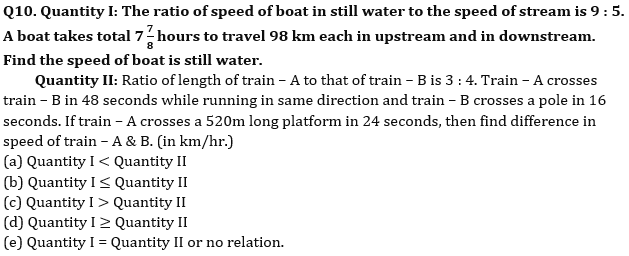Solutions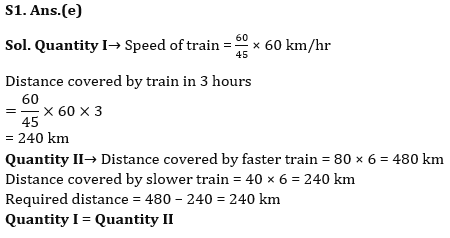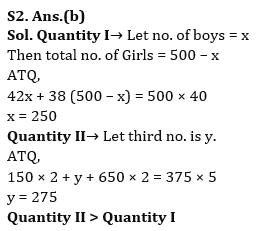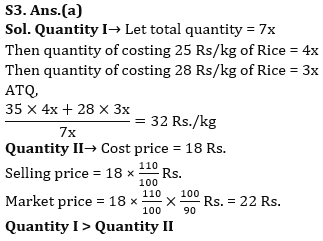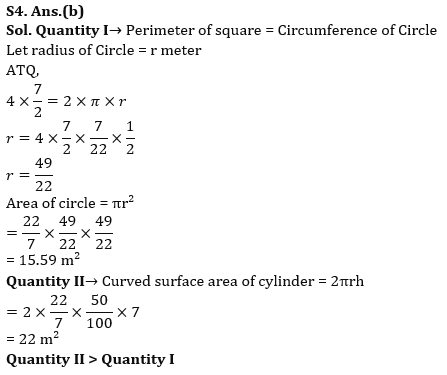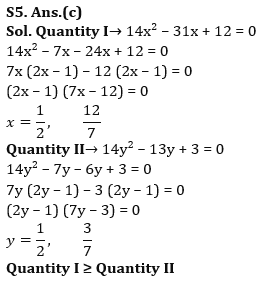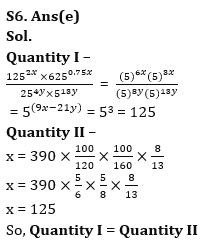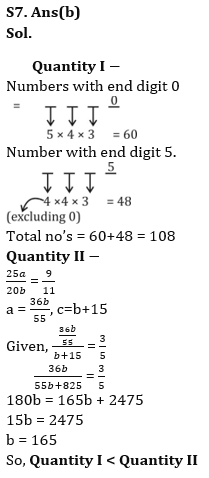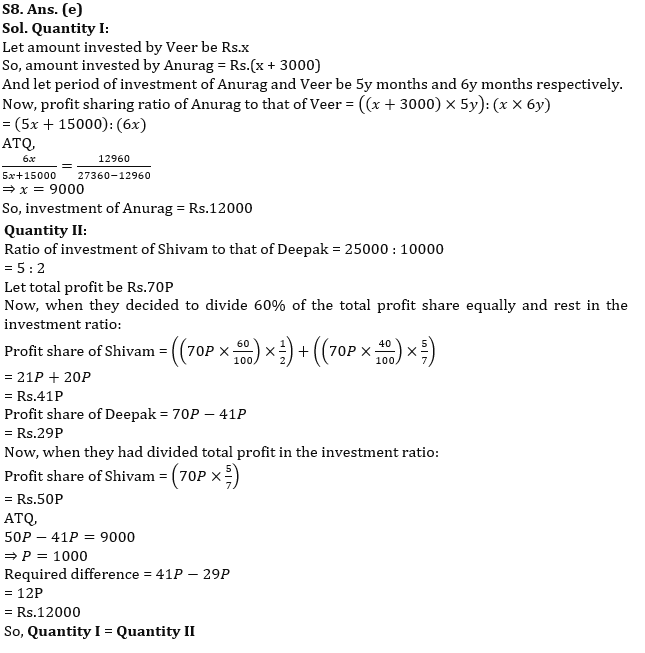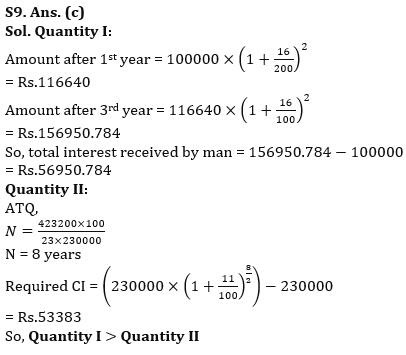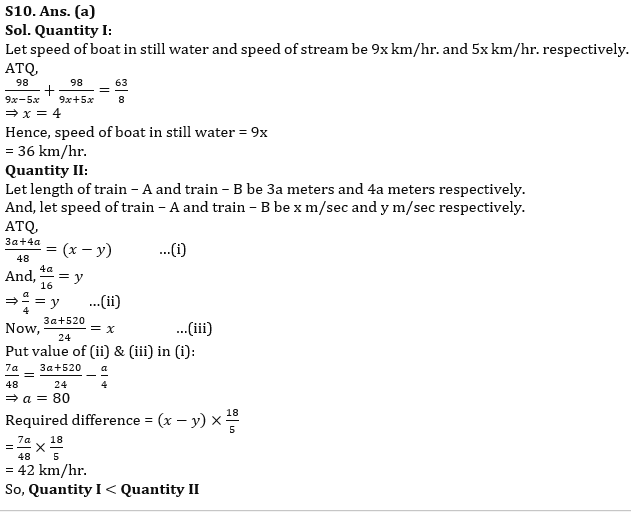## FAQs

### How many sections are there in the RBI Grade B Phase 1 Exam?

There are 4 sections in the RBI Grade B Phase 1 Exam i.e. English Language, General Awareness, Quantitative Aptitude & Reasoning.

#### Congratulations!Union Budget 2023-24: Free PDF